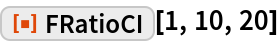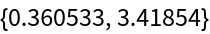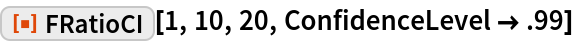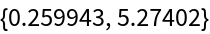#Function Repository Resource:

# FRatioCI

Estimate the confidence interval based on an F-ratio distribution

Contributed by: Wolfram Research
 ResourceFunction["FRatioCI"][ratio,n,m] gives a confidence interval based on an F-ratio distribution with n and m degrees of freedom.

## Details and Options

ResourceFunction["FRatioCI"][ratio,n,m] gives a confidence interval {min,max} for a ratio of variances estimated by ratio.
For confidence level α, min=ratio/q(1+α)/2 and max=ratio/q(1-α)/2 where qi is the ithquantile of an F-ratio distribution with n numerator and m denominator degrees of freedom.
ResourceFunction["FRatioCI"] is the confidence interval for a ratio of population variances based on sample variances estimated from samples of size n+1 and m+1.
The following option can be given:
 ConfidenceLevel 0.95 probability associated with a confidence interval

## Examples

### Basic Examples

A 95% confidence interval based on an F-ratio distribution:

 In:=Out=### Options

#### ConfidenceLevel

A 99% confidence interval:

 In:=Out=## Requirements

Wolfram Language 11.3 (March 2018) or above